## Capacitance of a parallel plate capacitor

• A parallel plate capacitor consists of two large plane parallel conducting plates separated by a small distance.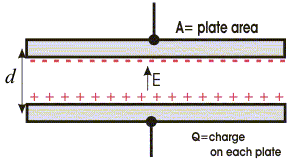• Suppose two plates of the capacitor have equal and opposite charge Q on them. If A is the area of each plate then surface charge density on each plate is
• Suppose two plates of the capacitor have equal and opposite charge Q on them. If A is the area of each plate then surface charge density on each plate is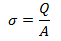• We have already calculated field between two oppositely charged plates using Gauss’s law which is and in this result effects near the edges of the plates have been neglected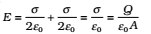• Since electric field between the plates is uniform the potential difference between the plates is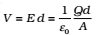where d is the separation between the plates.

• Thus, capacitance of parallel plate capacitor in vacuum is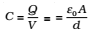• We see that quantity on which capacitance of parallel plate capacitor depends i.e.,ε0 , A and d are all constants for a capacitor.
• Thus we see that in this case capacitance is independent of charge on the capacitor but depends on the area of its plates and separation distance between the plates.